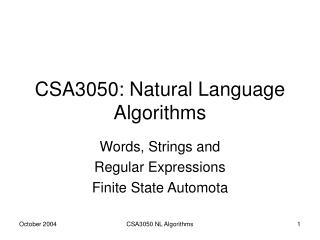DownloadDownload PresentationCSA3050: Natural Language Algorithms

# CSA3050: Natural Language Algorithms

Télécharger la présentation## CSA3050: Natural Language Algorithms

- - - - - - - - - - - - - - - - - - - - - - - - - - - E N D - - - - - - - - - - - - - - - - - - - - - - - - - - -
##### Presentation Transcript

1. CSA3050: Natural Language Algorithms Words, Strings and Regular Expressions Finite State Automota CSA3050 NL Algorithms

2. This lecture • Outline • Words • The language of words • FSAs in Prolog • Acknowledgement • Jurafsky and Martin, Speech and Language Processing, Prentice Hall 2000 • Blackburn and Steignitz: NLP Techiques in Prolog:http://www.coli.uni-sb.de/~kris/nlp-with-prolog/html/ CSA3050 NL Algorithms

3. What is a Word? • A series of speech sounds that symbolizes meaning without being divisible into smaller units • Any segment of written or printed discourse ordinarily appearing between spaces or between a space and a punctuation mark • A set of linguistic forms produced by combining a single base with various inflectional elements without change in the part-of-speech elements • A number of bytes processed as a unit. CSA3050 NL Algorithms

4. Information Associated with Words • Spelling • orthographic • phonological • Syntax • POS • Valency • Semantics • Meaning • Relationship to other words CSA3050 NL Algorithms

5. Properties of Words • Sequence • characters pollution • phonemes • Delimitation • whitespace • other? • Structure • simple ("atomic“) words • complex ("molecular") words CSA3050 NL Algorithms

6. Complex Words • enlargementen + large + ment(en + large) + menten + (large + ment) • affixation • prefix • suffix • infix CSA3050 NL Algorithms

7. Sets Underly the Formation of Complex Words prefixes roots suffixes large charge infect code decide ed ing ee er ly dis re un en + + CSA3050 NL Algorithms

8. Structure of Complex Words • Complex words are made by concatenating elements chosen from • a set of prefixes • a set of roots • a set of suffixes • The set of valid words for a given human language (e.g. English, Maltese) can be regarded as a formal language. CSA3050 NL Algorithms

9. The Language of Words • What kind of formal language is the language of words? • One which can be constructed out of • A characteristic set of basic symbols (alphabet) • A characteristic set of combining operations • Union (disjunction) • Concatenation • Closure (iteration) • Regular Language; Regular Sets CSA3050 NL Algorithms

10. MACHINE Characterising Classes of Set CLASS OF SETS or LANGUAGES NOTATION CSA3050 NL Algorithms

11. Regular Expressions • Notation for describing regular sets • Used extensively in the Unix operating system (grep, sed, etc.) and also in some Microsoft products (Word) • Xerox Finite State tools use a somewhat different notation, but similar function. CSA3050 NL Algorithms

12. Regular Expressions a a simple symbol A B concatenation A | B alternation operator A & B intersection operator A* Kleene star CSA3050 NL Algorithms

13. MACHINE Characterising Classes of Set CLASS OF SETS or LANGUAGES NOTATION CSA3050 NL Algorithms

14. Finite Automaton • A finite automaton comprises • A finite set of states Q • An alphabet of symbols I • A start state q0  Q • A set of final states F  Q • A transition function δ(q,i) which maps a state q  Q and a symbol i  I to a new state q'  Q CSA3050 NL Algorithms

15. Encoding FSAs in Prolog • Three predicates • initial/1initial(s) – s is an initial state • final/1final(f) – f is a final state • arc/3arc(s,t,c)there is an arc from s to t labelled c CSA3050 NL Algorithms

16. 1- h 2 a h 3 ! 4= Example 1: FSA initial(1).final(4).arc(1,2,h).arc(2,3,a).arc(3,4,!).arc(3,2,h). CSA3050 NL Algorithms

17. Example 2: FSA with jump arc initial(1).final(4).arc(1,2,h).arc(2,3,a).arc(3,4,!).arc(3,1,#). 1- h 2 a # 3 ! 4= CSA3050 NL Algorithms

18. Example 3: NDA initial(1).final(4).arc(1,2,h).arc(2,3,a).arc(3,4,!).arc(2,1,a). 1- a h 2 a 3 ! 4= CSA3050 NL Algorithms

19. A Recogniser recognize1(Node,[ ]) :-    final(Node). recognize1(Node1,String) :-    arc(Node1,Node2,Label),    traverse1(Label,String,NewString),    recognize1(Node2,NewString). traverse1(Label,[Label|Symbols],Symbols). CSA3050 NL Algorithms

20. Trace Call: (7) test1([h, a, !]) Call: (8) initial(_L181) Exit: (8) initial(1) Call: (8) recognize1(1, [h, a, !]) Call: (9) arc(1, _L199, _L200) Exit: (9) arc(1, 2, h) Call: (9) traverse1(h, [h, a, !], _L201) Exit: (9) traverse1(h, [h, a, !], [a, !]) Call: (9) recognize1(2, [a, !]) Call: (10) recognize1(3, [!]) Call: (11) recognize1(4, []) Call: (12) final(4) Exit: (12) final(4) Exit: (11) recognize1(4, []) Exit: (10) recognize1(3, [!]) Exit: (9) recognize1(2, [a, !]) Exit: (8) recognize1(1, [h, a, !]) Exit: (7) test1([h, a, !]) CSA3050 NL Algorithms

21. Generation • test1(X) • X = [h, a, !] ; • X = [h, a, h, a, !] ; • X = [h, a, h, a, h, a, !] ; • X = [h, a, h, a, h, a, h, a, !] ; • etc. CSA3050 NL Algorithms

22. FINITE STATE NETWORKS 3 Related Frameworks REGULAR LANGS/SETS describe recognise REGULAR EXPRESSIONS CSA3050 NL Algorithms

23. Regular Operations • Operations • Concatenation • Union • Closure • Over What • Language • Expressions • FS Automota CSA3050 NL Algorithms

24. Regular Expression E1: = [a|b] E2: = [c|d] E1 E2 = [a|b] [c|d] Language L1 = {"a", "b"} L2 = {"c", "d"} L1 L2 = {"ac", "ad", "bc", "bd"} Concatenation over Reg. Expression and Language CSA3050 NL Algorithms

25. Concatenation overFS Automata a c ⌣ b d a c = b d CSA3050 NL Algorithms

26. Issues • Handling jump arcs. • Handling non-determinism • Computing operations over networks. • Maintaining multiple states in DB • Representation. CSA3050 NL Algorithms